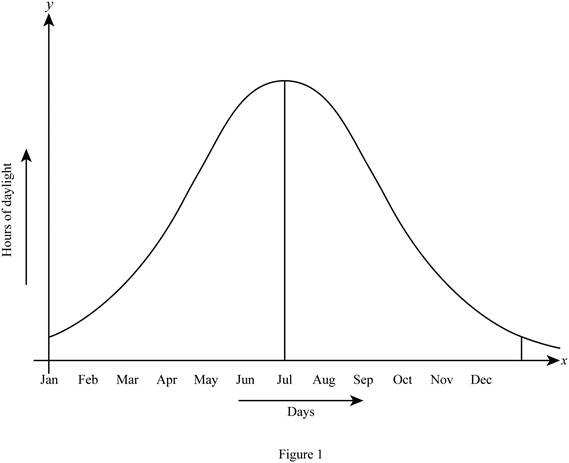# A rough graph of the number of hours of daylight as a function of the time of year.### Single Variable Calculus: Concepts...

4th Edition
James Stewart
Publisher: Cengage Learning
ISBN: 9781337687805### Single Variable Calculus: Concepts...

4th Edition
James Stewart
Publisher: Cengage Learning
ISBN: 9781337687805

#### Solutions

Chapter 1.1, Problem 14E
To determine

## To sketch: A rough graph of the number of hours of daylight as a function of the time of year.

Expert Solution

### Explanation of Solution

Let the time of year be represented in the x-axis and the number of hours of daylight be represented in the y-axis.

The day which has the maximum hours of daylight is known as the longest day of the year and the day which has the minimum hours of daylight is known as the shortest day of the year.

For the northern hemisphere, the longest day occurs in late June and the shortest day occurs in late December.

For the southern hemisphere, the days will be interchanged. That is, the longest day occurs in late December and the shortest day occurs in late June.

Thus, the rough graph of the number of hours of daylight as a function of the time of year based on the northern hemisphere is shown below in Figure 1.Here, the graph seems to be a bell-shaped curve.

### Have a homework question?

Subscribe to bartleby learn! Ask subject matter experts 30 homework questions each month. Plus, you’ll have access to millions of step-by-step textbook answers!